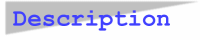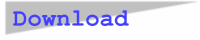### LinSat for JavaLinSat estimates four linearly transformable functions and two saturation models for trend extrapolation. It computes four goodness-of-fit criteria, viz. RMSE = Root Mean Squared Error, MAD = Mean Absolute Deviation, MAPE = Mean Absolute Percentage Error, and MPE = Mean Percentage Error or Bias. Forecast values may be calculated for each function of the user's choice. Observed and fitted data are plotted in a line graph. The demo data file Sample17.dat contains 17 datapoints.